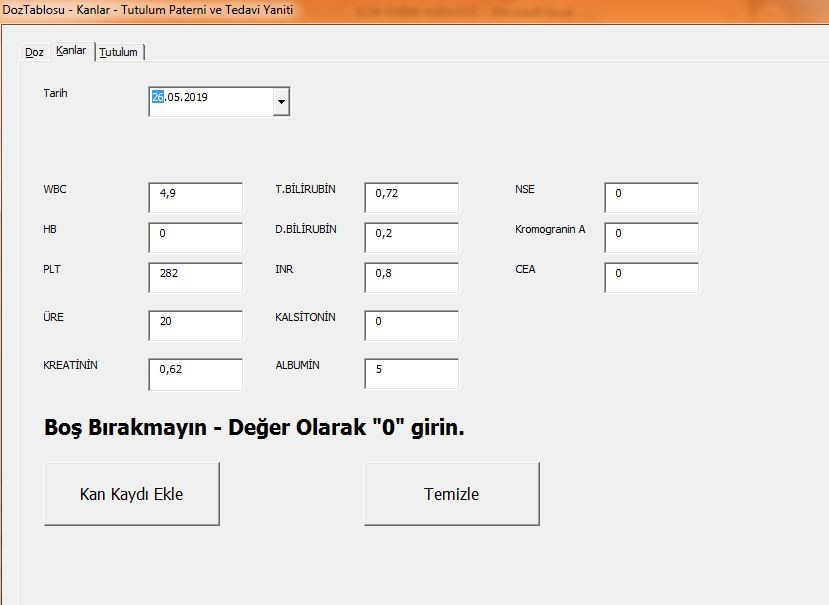# Thread: Charting Empty CellsThanks: 0Likes: 0

1. ##Charting Empty Cells

Hello
I provide some numbers via my excel userform to a sheet.
then I use that sheet to provide data to my charts.

Value of zero is a great problem. It makes my graphs meaningless.

how to solve this problem?

(userform pastes numbers to sheet)Reply With Quote

2. ##Re: Charting Empty Cells

Charts will skip N/A values, so if U adjust the range that your charting to replace zero with N/A then it should work...
Code:
`=If(A1=0,"N/A",A1)`
HTH. DaveReply With Quote

3. ##Re: Charting Empty Cells

But there is a problem.

My userform owerwrites the formula.
I use these cells to enter values from my userformCode:
```Private Sub submit1_Click()Dim ssheet1 As Worksheet
Set ssheet1 = ThisWorkbook.Sheets("Kanlar")

nr = ssheet1.Cells(Rows.count, 1).End(xlUp).Row + 1

ssheet1.Cells(nr, 1) = CDate(Me.DTpick1)
ssheet1.Cells(nr, 2) = CDec(Me.tbWBC)
ssheet1.Cells(nr, 3) = CDec(Me.tbHB)
ssheet1.Cells(nr, 4) = CDec(Me.tbPLT)
ssheet1.Cells(nr, 5) = CDec(Me.tbUREA)
ssheet1.Cells(nr, 6) = CDec(Me.tbKREA)
ssheet1.Cells(nr, 7) = CDec(Me.tbTBIL)
ssheet1.Cells(nr, 8) = CDec(Me.tbDBIL)
ssheet1.Cells(nr, 9) = CDec(Me.tbINR)
ssheet1.Cells(nr, 10) = CDec(Me.tbKALS)
ssheet1.Cells(nr, 11) = CDec(Me.tbALB)
ssheet1.Cells(nr, 12) = CDec(Me.tbNSE)
ssheet1.Cells(nr, 13) = CDec(Me.tbKROGA)
ssheet1.Cells(nr, 14) = CDec(Me.tbCEA)

Application.ScreenUpdating = True
Worksheets("Kanlar").Select```Reply With Quote

4. ##Re: Charting Empty Cells

Maybe this bit of code addendum..
Code:
```For cnt = 1 to 14
If ssheet1.Cells(nr, cnt) = 0 then
ssheet1.Cells(nr, cnt) = "N/A"
next cnt```
DaveReply With Quote

5. ##Re: Charting Empty CellsOriginally Posted by NdNoviceHlpMaybe this bit of code addendum..
Code:
```For cnt = 1 to 14
If ssheet1.Cells(nr, cnt) = 0 then
ssheet1.Cells(nr, cnt) = "N/A"
next cnt```
Dave

Code:
```Private Sub submit1_Click()Dim ssheet1 As Worksheet
Set ssheet1 = ThisWorkbook.Sheets("Kanlar")

nr = ssheet1.Cells(Rows.count, 1).End(xlUp).Row + 1

ssheet1.Cells(nr, 1) = CDate(Me.DTpick1)
ssheet1.Cells(nr, 2) = CDec(Me.tbWBC)
ssheet1.Cells(nr, 3) = CDec(Me.tbHB)
ssheet1.Cells(nr, 4) = CDec(Me.tbPLT)
ssheet1.Cells(nr, 5) = CDec(Me.tbUREA)
ssheet1.Cells(nr, 6) = CDec(Me.tbKREA)
ssheet1.Cells(nr, 7) = CDec(Me.tbTBIL)
ssheet1.Cells(nr, 8) = CDec(Me.tbDBIL)
ssheet1.Cells(nr, 9) = CDec(Me.tbINR)
ssheet1.Cells(nr, 10) = CDec(Me.tbKALS)
ssheet1.Cells(nr, 11) = CDec(Me.tbALB)
ssheet1.Cells(nr, 12) = CDec(Me.tbNSE)
ssheet1.Cells(nr, 13) = CDec(Me.tbKROGA)
ssheet1.Cells(nr, 14) = CDec(Me.tbCEA)

For cnt = 1 to 14
If ssheet1.Cells(nr, cnt) = 0 then
ssheet1.Cells(nr, cnt) = "N/A"
next cnt

Application.ScreenUpdating = True
Worksheets("Kanlar").Select

End Sub```

not working(Reply With Quote

6. ##Re: Charting Empty Cells

The great Google seems to indicate that this line of code should be..
Code:
`ssheet1.Cells(nr, cnt) = NA()`
Maybe that will work? DaveReply With Quote

7. ##Re: Charting Empty Cells

Code:
```Private Sub submit1_Click()Dim ssheet1 As Worksheet
Set ssheet1 = ThisWorkbook.Sheets("Kanlar")

nr = ssheet1.Cells(Rows.count, 1).End(xlUp).Row + 1

ssheet1.Cells(nr, 1) = CDate(Me.DTpick1)
ssheet1.Cells(nr, 2) = CDec(Me.tbWBC)
ssheet1.Cells(nr, 3) = CDec(Me.tbHB)
ssheet1.Cells(nr, 4) = CDec(Me.tbPLT)
ssheet1.Cells(nr, 5) = CDec(Me.tbUREA)
ssheet1.Cells(nr, 6) = CDec(Me.tbKREA)
ssheet1.Cells(nr, 7) = CDec(Me.tbTBIL)
ssheet1.Cells(nr, 8) = CDec(Me.tbDBIL)
ssheet1.Cells(nr, 9) = CDec(Me.tbINR)
ssheet1.Cells(nr, 10) = CDec(Me.tbKALS)
ssheet1.Cells(nr, 11) = CDec(Me.tbALB)
ssheet1.Cells(nr, 12) = CDec(Me.tbNSE)
ssheet1.Cells(nr, 13) = CDec(Me.tbKROGA)
ssheet1.Cells(nr, 14) = CDec(Me.tbCEA)

For nInputRow = 1 To 14
If ssheet1.Cells(nr, nInputRow) = 0 Then
ssheet1.Cells(nr, nInputRow) = "#YOK"
End If
Next nInputRow

Application.ScreenUpdating = True
Worksheets("Kanlar").Select

End Sub```

now it works.
we forgot to add "end if"Reply With Quote

8. ##Re: Charting Empty Cells

I am trying to plot a chart that may sometimes contain blank values for the chart area. When I go to change the setting to "show gaps for empty cells", the option is grayed out. Why?Reply With Quote

9. ##Re: Charting Empty Cells

Well secoo140 I'm not sure about the "we" in #7 ? Maybe start a new post re. what's up with your previous post? Happy to have helped U out. DaveReply With Quote

## User Tag List

#### Tags for this Thread

numbers, problem, provide, sheet, userform####Posting Permissions

• You may not post new threads
• You may not post replies
• You may not post attachments
• You may not edit your posts
•